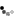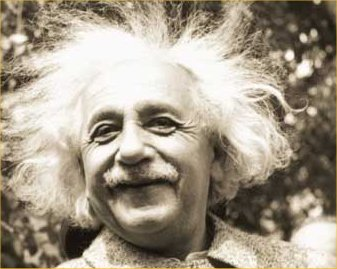Loading... Please wait...

## E = mc²In physics, mass–energy equivalence is the concept that the mass of a body is a measure of its energy content. In this concept the total internal energy E of a body at rest is equal to the product of its rest mass m and a suitable conversion factor to transform from units of mass to units of energy. If the body is not stationary relative to the observer then account must be made for relativistic effects where m is given by the relativistic mass and E the relativistic energy of the body.

Albert Einstein proposed mass–energy equivalence in 1905 in one of his Annus Mirabilis papers entitled "Does the inertia of a body depend upon its energy-content?" The equivalence is described by the famous equationwhere E is energy, m is mass, and c is the speed of light in a vacuum. The formula does not depend on any specific system of measurement units. For example, in natural units, the speed of light is set equal to 1, and the formula becomes the identity E = m; hence the term mass–energy equivalence.

The equation E = mc2 indicates that energy always exhibits mass in whatever form the energy takes. It does not imply that mass may be “converted” to energy, for modern theory holds that neither mass nor energy may be destroyed, but only moved from one location to another. In physics, mass must be differentiated from matter. In cases where matter particles are created or destroyed, the precursors and products retain both the original mass and energy, which is unchanged. Mass–energy equivalence also means that mass conservation becomes a restatement of the law of energy conservation, which is the first law of thermodynamics.

Einstein was not the first to propose a mass–energy relationship.However, Einstein was the first scientist to propose the E = mc2 formula and the first to interpret mass-energy equivalence as a fundamental principle that follows from the relativistic symmetries of space and time.

Source: Wikipedia® is a registered trademark of the Wikimedia Foundation, Inc., a non-profit organization.

"Why is it that nobody understands me and everybody likes me?"